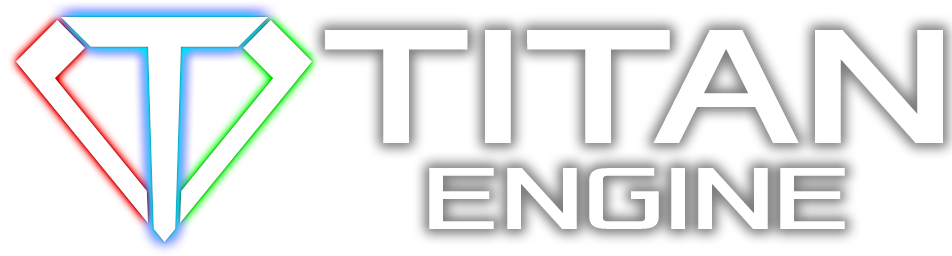About Store Forum Documentation ContactPhysics not moving along with car mesh Author Message
OtoloneMember

 Post: #1 Physics not moving along with car mesh Hi there! I followed Scarlet thread's tutorial on Game Object basics. But I realized that my Car object's physics is stationary when I move the car. Which implies that my car moves through walls.How do I update the physics so that it moves simultaneously with the car mesh? Thanks in advance.Here is the code. Code: ```class Car : public Game::Obj {    public:    Vec dir;    Car ::Car();    Car::~Car();       //draw     UInt drawPrepare();         void drawShadow();       void create(Object &obj);       virtual Vec    pos         (                ){return _matrix.pos;} // get position    virtual void   pos         (C Vec    &pos   ){_matrix.pos = pos; _scaled_matrix.pos=pos;}// set position    virtual Matrix matrix      (                ){return _matrix;} // get matrix    virtual Matrix matrixScaled(          ){return _scaled_matrix;} // get the scale matrix.Not found in Game::Obj    virtual void   matrix(C Matrix &matrix){_matrix = matrix; _scaled_matrix = matrix; _scaled_matrix.scaleOrnL(scale);} // set matrix          // get    bool InSpawnRange(Player&chr)    {       return Dist(pos(), chr.pos()) <= 15;           }          // move    void move()    {       // code to move car         }    // update     Bool  update(); // update, return false when object wants to be deleted, and true if wants to exist    //       private: Matrix _matrix, _scaled_matrix; Vec scale; MeshPtr mesh; MaterialPtr material; PhysBodyPtr phys; Actor  actor; PathObstacle obst; Controller ctr;    float    range; float    damage; float    fuel; } Car ::Car() : range(7.0f), damage(10.0f), fuel(100.0f){}; Car ::~Car() {    } void Car::create(Object &obj) { scale      = obj.scale3(); mesh       = obj.mesh(); phys        = obj.phys(); _matrix     = obj.matrixFinal().normalize();   _scaled_matrix = _matrix;   _scaled_matrix.scaleOrnL(scale);      dir = matrix().orn().z;      if(phys) {    actor.create(*phys, 1, scale, true).matrix(_matrix).collision(true);    actor.obj(this);    actor.group(AG_DEFAULT);    ctr.create(Capsule(0.5, 0.4, pos()));    }    } Bool Car::update() {            move();    ctr.update(pos(), false, false);       return true; } uint Car::drawPrepare() {    if(mesh && Frustum(mesh->ext, _scaled_matrix))    {          mesh->draw(_scaled_matrix);           }    uint modes = scale.length();    return modes; } void  Car::drawShadow() {    if(mesh && Frustum(mesh->ext, _scaled_matrix))    {          mesh->draw(_scaled_matrix);           } }``` Please how do improve the code? Below is a screenshot of the situation. Attached File(s) Image(s)(This post was last modified: 01-31-2019 02:57 AM by Otolone.)
01-31-2019 02:53 AM
 « Next Oldest | Next Newest »